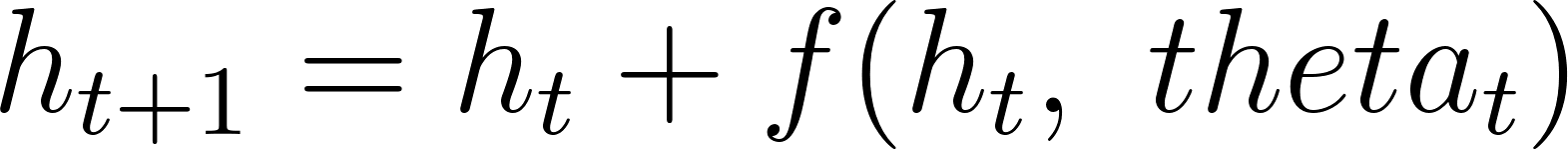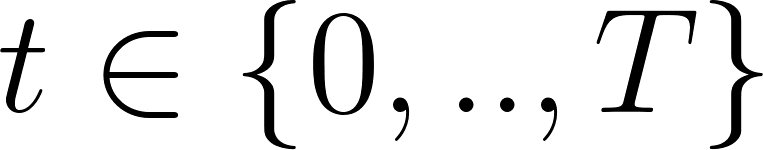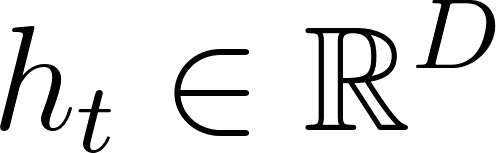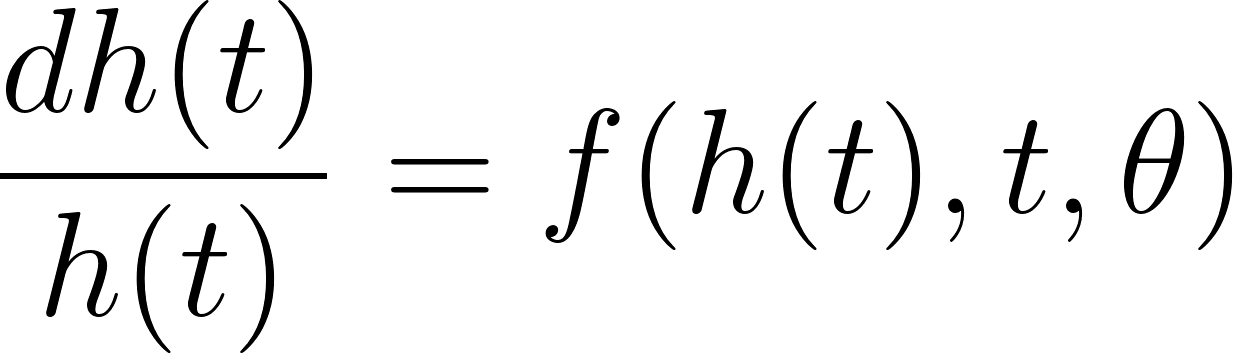• A
• A
• A
• АБВ
• АБВ
• АБВ
• А
• А
• А
• А
• А
Обычная версия сайта

# Заседание научного семинара лаборатории ЛАТАС

Мероприятие завершено

Дата и время: 22 ноября в 16:00
Язык: английский
Спикер: Alexander Ponomarenko (lab LATNA, HSE NN)
Тема: Neural Ordinary Differential Equation and Application To Establishing Parameters Of Atmospheric Thermal Radiation
Формат: онлайн на платформе Zoom

Models such as residual networks, recurrent neural network decod-ers, and normalizing flows build complicated transformations by composing a sequence of transformations to a hidden state:, whereand.These iterative updates can be seen as an Euler discretization of a continuous transformation. What happens as we add more layers and take smaller steps? This idea is a foundation of Neu-ral Ordinary Differential Equation (NeuralODE), which parameterized the con-tinuous dynamics of hidden units using an ordinary differential equation speci-fied by a neural network.

NeuralODE is a powerful tool for dealing with real physical processes, where data is naturally consistent and continuous, in the sense that the data points are observations of a continuity changing state. As a demonstration of the power of the NeuralODE method we will consider a problem of establishing parameters of atmospheric thermal radiation.

Приглашаются все желающие!
Явка аспирантов школы компьютерных наук обязательна.

Для участия, пожалуйста, зарегистрируйтесь